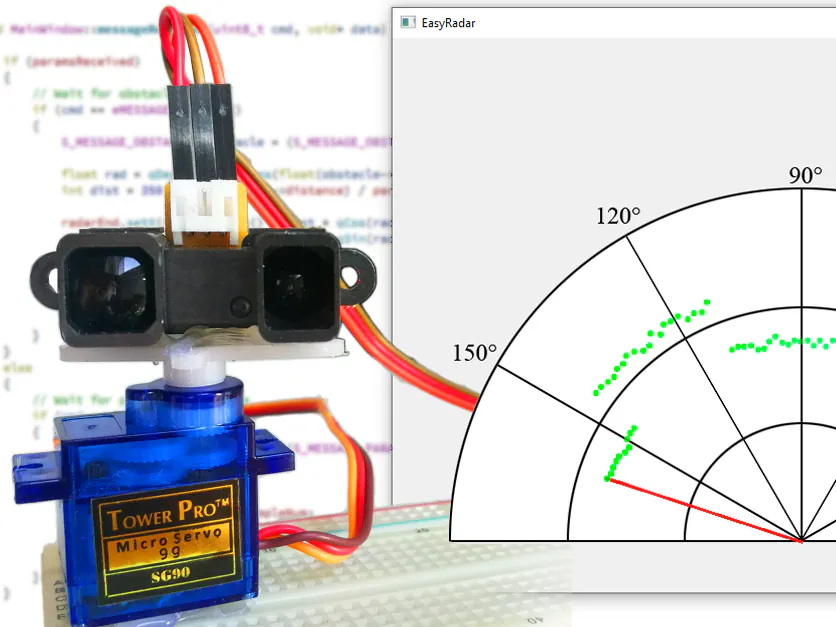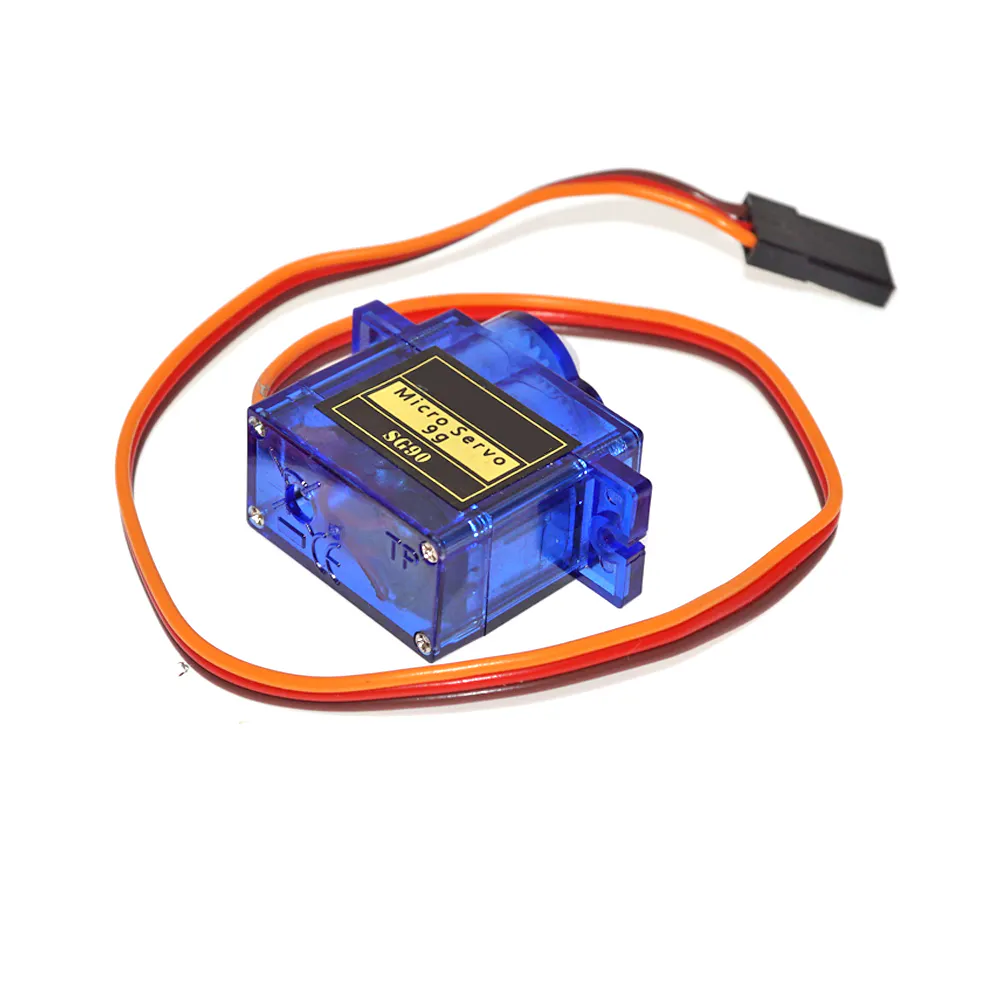# Infrared Radar with Sharp Distance Sensor

In this small project I show you how to create a radar system using Arduino, Sharp Infrared distance sensors and a micro servo.

IntermediateFull instructions provided949## Things used in this project

### Hardware componentsSG90 Micro-servo motor
×1
 SparkFun Infrared Proximity Sensor
×1

### Software apps and online servicesArduino IDE

## Code

Arduino
You can customize some paremeters at the top of the document with macros.
Note, that if you change the characteristic of the Sharp distance sensor, you have to modify distAdcMap[] array values!
```#include <Servo.h>

// ------------------------------------------------------------
// User parameters
// ------------------------------------------------------------
// Define pin where Sharp sensor is located.
#define SHARP_PIN       PA0
// Define pin where servo is located.
#define SERVO_PIN       PA9
// Define the maximum distance you want to measure in [cm].
#define DISTANCE_MAX    150
// Define the angle value that servo will step between measurements.
#define SERVO_STEP      2
// Define the delay in [ms] between measurements.
#define MEAS_DELAY_MS   30

// ------------------------------------------------------------
// Message handling
// ------------------------------------------------------------
// Sync value in message header
#define MSG_SYNC                (0x55AA)
// Start of checksum calculation in message header
#define MSG_CHECKSUM_START      (0x55)
// Maximum size of a message
#define MSG_MAX_SIZE            (64)

typedef struct
{
uint16_t sync;
uint8_t checkSum;
uint8_t cmd;

// Message: Parameters
typedef struct
{
uint16_t distanceMax;
uint16_t sampleNum;
} S_MESSAGE_PARAMETERS;

// Message: Obstacle
typedef struct
{
uint16_t angle;
uint16_t distance;
} S_MESSAGE_OBSTACLE;

// Message identifiers
typedef enum
{
eMESSAGE_PARAMETERS,
eMESSAGE_OBSTACLE,
} E_MESSAGE_CMD;

// Function to send a message to PC Application
void sendMessage(uint8_t cmd, void* data)
{
// Structure for message to send
static struct
{
uint8_t data[MSG_MAX_SIZE];
} sendData;
uint8_t msgSize;

// Get size of data based on command
switch (cmd)
{
case eMESSAGE_PARAMETERS:
msgSize = sizeof(S_MESSAGE_PARAMETERS);
break;
case eMESSAGE_OBSTACLE:
msgSize = sizeof(S_MESSAGE_OBSTACLE);
break;
}

// Fill data bytes
for (uint8_t i = 0; i < msgSize; i++)
{
sendData.data[i] = ((uint8_t*)(data))[i];
}

// Send composed message to PC
}

// ------------------------------------------------------------
// Distance calculation
// ------------------------------------------------------------
// Structure to store ADC Value - Distance pairs
typedef struct {
float distance;

// TODO
// ADC Value - Distance pairs
// Fill it measured SHARP Sensor characteristic values.
{12,  3599},
{20,  3122},
{30,  2295},
{40,  1733},
{50,  1381},
{60,  1191},
{70,  1000},
{80,  855},
{90,  751},
{100, 662},
{110, 606},
{120, 559},
{130, 517},
{140, 465},
{150, 418},
};

// Function to get distance [cm] from measured ADC Value
{
float distance = DISTANCE_MAX;

// Linear interpolation from measured ADC value and MAP.
{
{
break;
}
}

return distance;
}

// ------------------------------------------------------------
// Application
// ------------------------------------------------------------
// Global variables
Servo myServo;
int servoVal = 0;
int servoDir = SERVO_STEP;
S_MESSAGE_OBSTACLE msgObstacle;
S_MESSAGE_PARAMETERS msgParameters;

void setup()
{
Serial.begin(115200);
myServo.attach(SERVO_PIN);
myServo.write(servoVal);

msgParameters.distanceMax = DISTANCE_MAX;
msgParameters.sampleNum = (180/SERVO_STEP);
}

void loop()
{
float distance;

// Send parameters at start
if (servoVal == 0)
sendMessage(eMESSAGE_PARAMETERS, &msgParameters);

// Read sharp sensor analog value and use a simple filter on it.
for (int i = 0; i < 10; i++)

// Compose send data structure from measured values
msgObstacle.angle    = servoVal;
msgObstacle.distance = (uint16_t)distance;
sendMessage(eMESSAGE_OBSTACLE, &msgObstacle);

// Set servo to next position
if (servoVal >= 180)
servoDir = -SERVO_STEP;
else if (servoVal <= 0)
servoDir = +SERVO_STEP;

servoVal += servoDir;
myServo.write(servoVal);

// Wait until new measurement
delay(MEAS_DELAY_MS);
}
```

### Characteristic Measurement

Arduino
Simple code to measure characteristic of Sharp distance sensor. Open serial communication to check measured ADC Values.
```void setup()
{
Serial.begin(115200);
}

void loop()
{

// Read sharp sensor analog value and use a simple filter on it
for (int i = 0; i < 50; i++)
{
delay(10);
}